• ## Python去极值方法

千次阅读 2021-01-11 16:18:52
#MAD:中位数去极值 def filter_extreme_MAD(series,n=5): median = series.quantile(0.5) new_median = ((series - median).abs()).quantile(0.50) max_range = median + n*new_median min_range = median - n*new_...


文章目录

import numpy as np
import pandas as pd
import matplotlib.pyplot as plt

# 构造测试数据
mean = 0.6
sigma = 1
num = 3500
np.random.seed(0)
factor_data = np.random.normal(mean, sigma, num)
factor_data = pd.Series(data=factor_data)
factor_data.index = [str(x).zfill(6) for x in factor_data.index.values]


def winsorize_plot(data, vertical_lines=[]):
fig = plt.figure(figsize=(12,6))
_df = ax.hist(data, 100,density=True)
color_list = ['red','green','yellow','black','gold','gray']
count = 0
for value in vertical_lines:
ax.bar(value, 0.1, width=0.05, color=color_list[count], alpha=1)
count += 1
plt.show()


data_mean = factor_data.mean()
std = np.std(factor_data)
std_up = data_mean+3*std
std_low = data_mean-3*std
quantile_low = np.percentile(factor_data, 2.5)
quantile_high = np.percentile(factor_data, 97.5)
vertical_lines = [std_up, std_low, quantile_high, quantile_low]
winsorize_plot(factor_data,vertical_lines)处理的逻辑：
第一步，找出所有因子的中位数

X

_

m

e

d

i

a

n

X\_median

第二步：得到每个因子与中位数的绝对偏差值

A

B

S

(

X

i

−

X

_

m

e

d

i

a

n

)

ABS(X_i-X\_median)

[

X

_

m

e

d

i

a

n

−

n

∗

M

A

D

,

X

_

m

e

d

i

a

n

+

n

∗

M

A

D

]

，并针对超出合理范围的因子值做如下的调整超出最大值的用最大值代替，小于最小值的用最小值代替。
#MAD:中位数去极值
median = series.quantile(0.5)
new_median = ((series - median).abs()).quantile(0.50)
max_range = median + n*new_median
min_range = median - n*new_median
return np.clip(series,min_range,max_range)


-1.417957718452538 2.54924039183218553σ法

3

σ

3\sigma

法又称为标准差法。标准差本身可以体现因子的离散程度，是基于因子的平均值

X

_

m

e

a

n

X\_mean

而定的。在离群值处理过程中，可通过用

X

_

m

e

a

n

±

n

∗

σ

X\_mean±n*\sigma

来衡量因子与平均值的距离。
第一步：计算出因子的平均值与标准差第二步：确认参数 n（这里选定 n = 3）第三步：确认因子值的合理范围为

[

X

_

m

e

d

i

a

n

−

n

∗

σ

,

X

_

m

e

d

i

a

n

+

n

∗

σ

]

[X\_median-n*\sigma,X\_median+n*\sigma]

，并对因子值作如下的调整：超出最大值的用最大值代替，小于最小值的用最小值代替。
# 3 sigma
def filter_extreme_3sigma(data,n=3,times=3):
# times进行times次3sigma处理
series = data.copy()
for i in range(times):
mean = series.mean()
std = series.std()
max_range = mean + n*std
min_range = mean - n*std
series = np.clip(series,min_range,max_range)
return series

sigma_winsorize = filter_extreme_3sigma(factor_data,3,3)
print (sigma_winsorize.min(),sigma_winsorize.max())
winsorize_plot(sigma_winsorize,vertical_lines)


-2.357313193311948 3.498196906137111百分位法
将因子值进行升序的排序，对排位百分位高于97.5%或排位百分位低于2.5%的因子值，进行类似于

M

A

D

、

3

σ

的方法进行调整。
#百分位法
def filter_extreme_percentile(series,min =0.025,max = 0.975):
series = series.sort_values()
q = series.quantile([min,max])
return np.clip(series,q.iloc,q.iloc)

percentile_winsorize = filter_extreme_percentile(factor_data,0.025,0.975)
print (percentile_winsorize.min(),percentile_winsorize.max())
winsorize_plot(percentile_winsorize,vertical_lines)


-1.2758881404314582 2.509630292141858引用自 --> 数据挖掘学习之路四：Python去极值方法
展开全文python 机器学习 数据分析
• 因子数据处理之去极值. 因子数据去极值的三种方法: 分位数去极值, 中位数绝对偏差去极值, 正态分布去极值 (不推荐).


第十课 因子数据处理之去极值
概述因子 Panel 结构分析截面数据序列数据
因子去极值分位数去极值中位数四分位数百分位数原理分析代码实现
中位数绝对偏差去极值计算方法代码实现
正态分布去极值代码实现
总结

概述
在我们构建策略之前首先要对得到的数据进行预处理. 去极值就是排除一些极端值的干扰. 如图: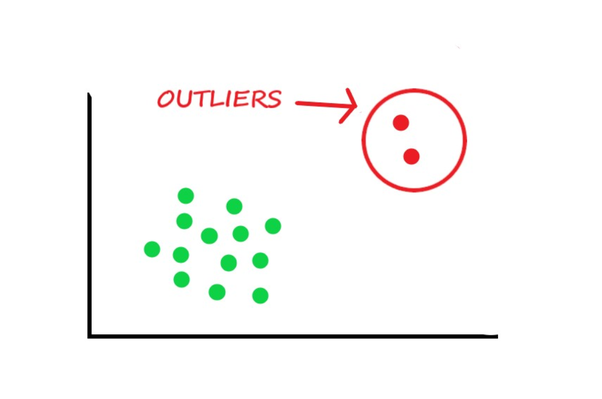因子 Panel 结构分析
Pandas 当中面板数据结构是三维的结构. 由截面数据和序列数据组成.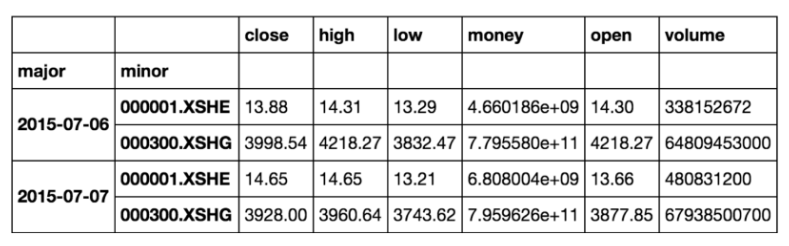代码:
get_price("000001.XSHE", start_date="2020-01-01", end_date="2020-01-06")

输出结果: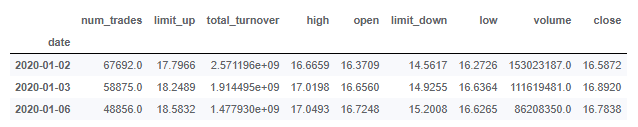price = get_price(["000024.XSHE","000001.XSHE","000002.XSHE"], start_date="2015-04-01",end_date="2015-04-12")
price

输出结果: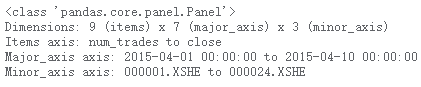截面数据
截面数据: 在同一时间, 不同统计单位相同统计指标组成的数据列.
# 获取个别财务数据
q = query(
fundamentals.income_statement.revenue,
fundamentals.income_statement.cost_of_goods_sold
).filter(
fundamentals.stockcode.in_(["000024.XSHE","000001.XSHE"])
)

fund = get_fundamentals(q, entry_date="2020-01-03")

# 换成截面
fund.iloc[:,0,:]

输出结果: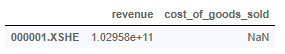# 获取所有财务数据
q = query(
fundamentals.income_statement.revenue,
fundamentals.income_statement.cost_of_goods_sold
)

fund = get_fundamentals(q, entry_date="2020-01-03")

# 换成截面
fund.iloc[:,0,:]

输出结果: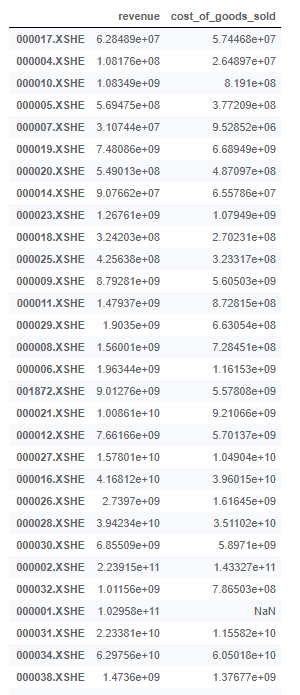序列数据
序列数据: 在不同时间点上收集到的数据. 这类数据反映了某一事物, 现象等随时间变的化状态或程度.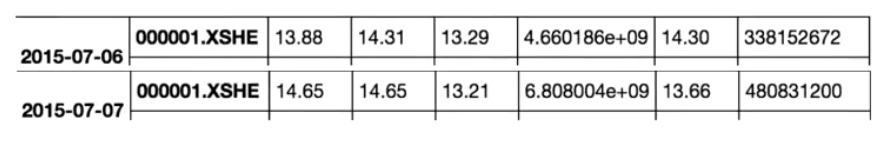注: 多因子分析使用的是截面数据而不是序列数据.
因子去极值
首先我们需要明白, 去极值不是删除 “异常数据”, 而是将这些数据 “拉回” 到正常的值.
去极值分为三种方法:
分位数去极值中位数绝对偏差去极值正态分布去极值
分位数去极值
首先我们来了解几个概念:
中位数四分位数百分位数
中位数
中位数是指将数据按大小顺序排列起来, 形成一个数列. 中位数就是居于数列中间位置的那个数. 中位数用 Me (Median 简写) 表示.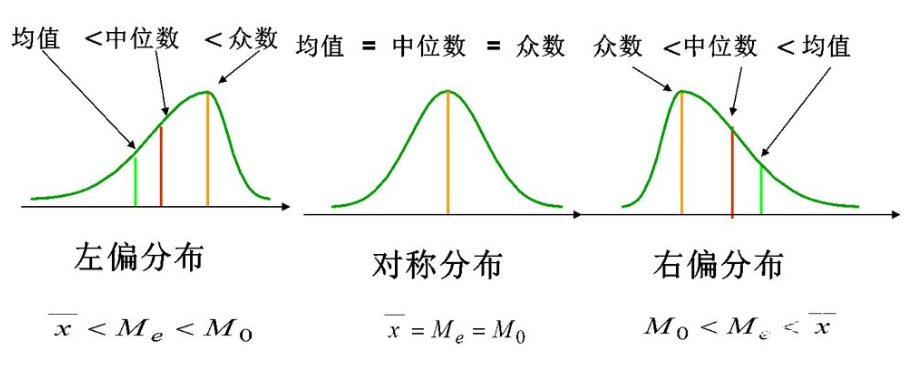从上图我们可以看出中位数相较于平均数受极大值 / 极小值的影响较小. 所以我们选择中位数而不是平均数.
四分位数
四分位数即把所有数值由小到大排列并分成四等分. 处于三个分割点位置的数值就是四分位数.第一四分位数 (Q1), 又称 “较小四分位数”, 等于该样本中所有数值由小到大排列后第 25% 的数字第二四分位数 (Q2), 又称 “中位数”, 等于该样本中所有的数值由小到大排序后第 50% 的数字第三四分位数 (Q3), 又称 “较大四分位数”, 等于该样本中所有的数值由小到大排序后第 75% 的数字
百分位数
百分位数即数据所处位置为整体的某个 % 位数. 关于百分位数有两种称呼, quantile 和 percentile.
原理
分位数去极值的原理是将指定分位数区间以外的极值用分位点的值替换掉.
分析
获取指定某个日期或者区间段的 pe_ratio 截面数据分位数去极值去极值结果与极值前结果比较
代码实现
函数:
import numpy as np

# 求出两个分位数的点的值
def quantile(factor, up, down):
"""分位数去极值"""
up_scale = np.percentile(factor, up)
down_scale = np.percentile(factor, down)

factor = np.where(factor > up_scale, up_scale, factor)
factor = np.where(factor < down_scale, down_scale, factor)
return factor

代码:
# 筛选条件
q = query(
fundamentals.eod_derivative_indicator.pe_ratio
)

# 获取数据
fund = get_fundamentals(q, entry_date="20200102").iloc[:, 0, :]

# 上下 2.5% 处理
fund["pe_ratio_fixed"] = quantile(fund["pe_ratio"], 97.5, 2.5)

# 画图
plt.figure(figsize=(15, 10))
plt.bar(fund.index[:500],fund['pe_ratio'][:500])
plt.bar(fund.index[:500],fund['pe_ratio_fixed'][:500])
plt.xticks(fund.index[:501:50])
plt.title("pe_ratio vs pe_ratio_fixed")
plt.show()

输出结果: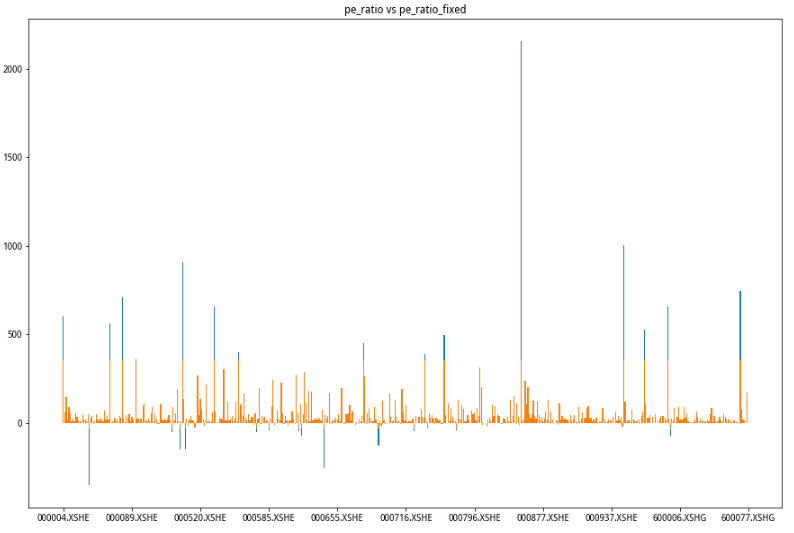中位数绝对偏差去极值
绝对偏差法 (MAD: Median Absolute Deviation) 是一种先需计算所有因子与中位数之间的距离综合来检测离群值的方法.
计算方法
去极值判断: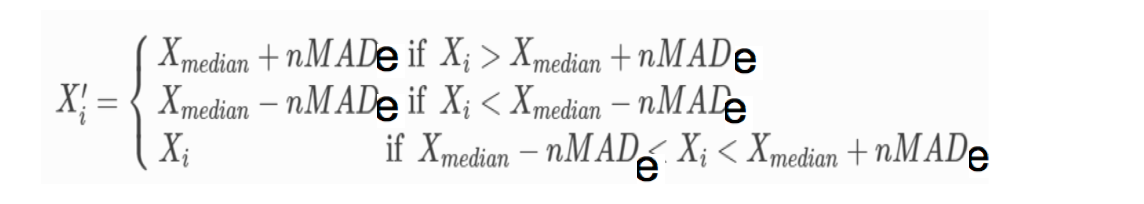注: 通常把偏移中位数三倍设为 MAD_e. 如果样本满足正态分布且足够大, 我们可以证明超过上下限的值为异常值.
代码实现
函数:
def mad(factor):
"""3倍中位数去极值"""

# 求出因子值的中位数
median = np.median(factor)

# 求出因子值与中位数的差值, 进行绝对值

# 定义几倍的中位数上下限
high = median + (3 * 1.4826 * mad)
low = median - (3 * 1.4826 * mad)

# 替换上下限
factor = np.where(factor > high, high, factor)
factor = np.where(factor < low, low, factor)
return factor

代码:
# 筛选条件
q = query(
fundamentals.eod_derivative_indicator.pe_ratio
)
# 获取数据
fund = get_fundamentals(q, entry_date="20200102").iloc[:, 0, :]

# 中位数绝对偏差法

# 画图
plt.figure(figsize=(15, 10))
plt.bar(fund.index[:500],fund['pe_ratio'][:500], color="green")
plt.bar(fund.index[:500],fund['pe_ratio_fixed'][:500])
plt.xticks(fund.index[:501:50])
plt.title("pe_ratio vs pe_ratio_fixed")
plt.show()

输出结果: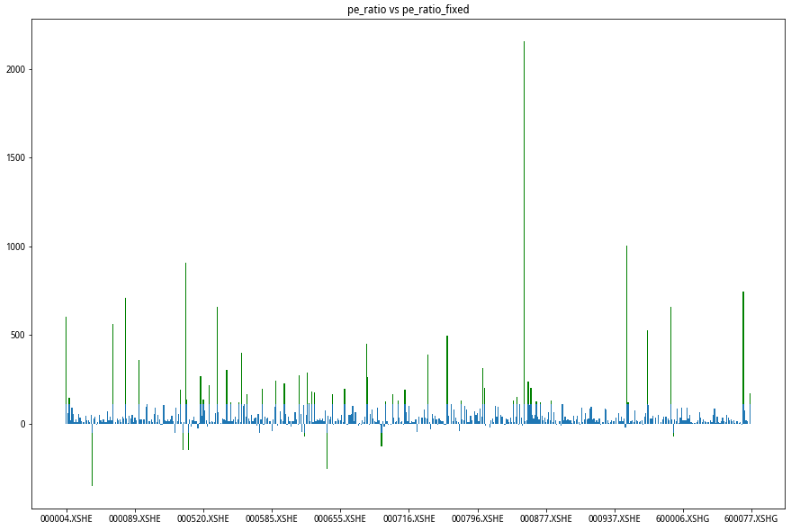正态分布去极值
正态分布 (Normal Distribution):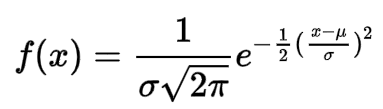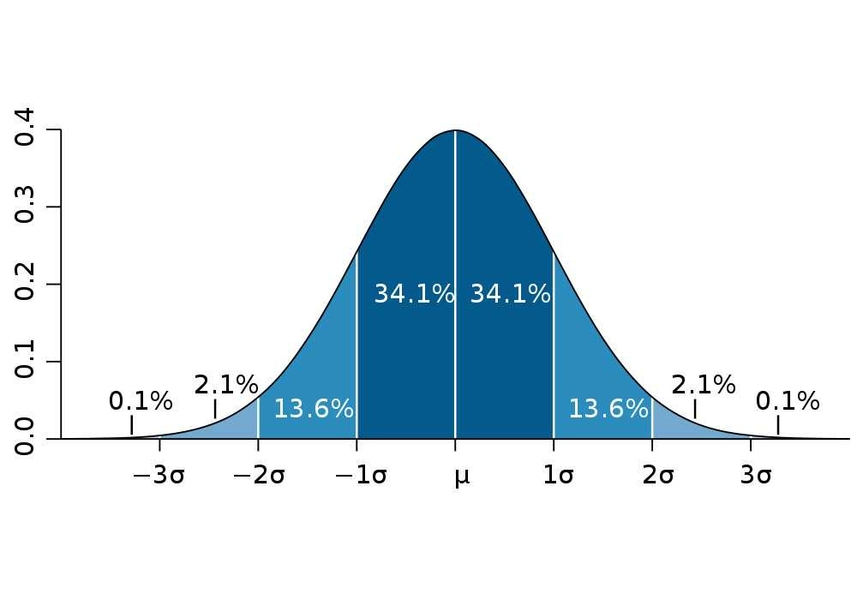1σ: 68.27% 的数据落在一个标准差之内2σ: 95.45% 的数据落在两个标准差之内3σ: 99.73% 的数据落在三个标准差之内4σ: 99.99% 的数据落在四个标准差之内
代码实现
def three_sigma(factor):
"""3 sigma 去极值"""

# 求出因子数据的平均数和标准差
mean = factor.mean()
std = factor.std()

# 左右的数据加减 3 个标准差
high = mean + (3 * std)
low = mean - (3 * std)

# 替换极值数据
factor = np.where(factor > high, high, factor)
factor = np.where(factor <low, low, factor)

return factor

# 筛选条件
q = query(
fundamentals.eod_derivative_indicator.pe_ratio
)
# 获取数据
fund = get_fundamentals(q, entry_date="20200102").iloc[:, 0, :]

# 3 sigma 去极值
fund["pe_ratio_fixed"] = three_sigma(fund["pe_ratio"])

# 画图
plt.figure(figsize=(15, 10))
plt.bar(fund.index[:500],fund['pe_ratio'][:500], color="orange")
plt.bar(fund.index[:500],fund['pe_ratio_fixed'][:500], color= "red")
plt.xticks(fund.index[:501:50])
plt.title("pe_ratio vs pe_ratio_fixed")
plt.show()

输出结果: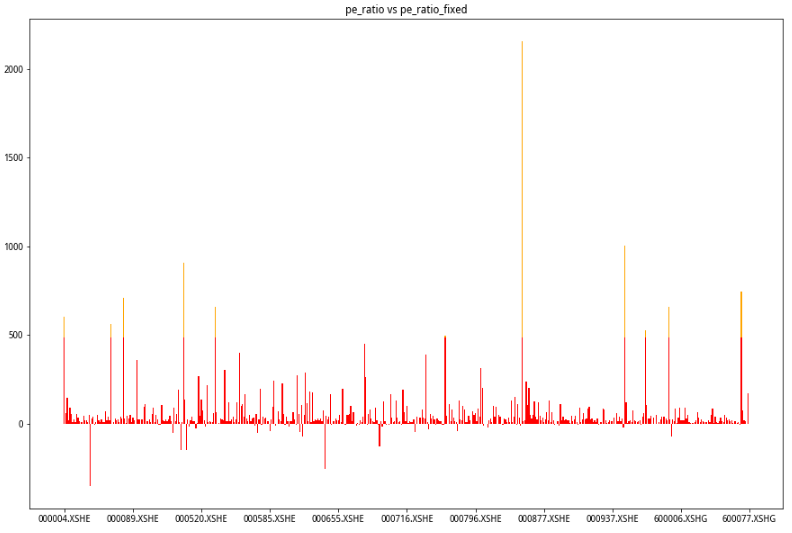总结
在做去极值的时候最好不要用正态分布去极值法, 推荐使用分位数和中位数绝对偏差去极值.
展开全文• 对于量化研究而言，拿到一份基础数据，首先需要对数据做预处理，以便于更好的探究数据规律，基于不同目标有不同处理环节，其中去极值、标准化、中性化这三点经常被放在一起讨论。整理网络资料，理解如下。 去极值 ...
对于量化研究而言，拿到一份基础数据，首先需要对数据做预处理，以便于更好的探究数据规律，基于不同目标有不同处理环节，其中去极值、标准化、中性化这三点经常被放在一起讨论。整理网络资料，理解如下。

3

σ

3\sigma

法、百分位法。
计算所有因子的中位数

X

m

e

d

i

a

n

X_{median}

计算每个因子与中位数的绝对偏差值

X

i

−

X

m

e

d

i

a

n

X_i-X_{median}

计算绝对偏差值的中位数

M

A

D

确定参数

n

n

，从而确定合理的范围为

[

X

m

e

d

i

a

n

−

n

∗

M

A

D

,

X

m

e

d

i

a

n

+

n

∗

M

A

D

]

，针对超出合理范围的值做如下调整：

X

i

′

=

{

X

m

e

d

i

a

n

+

n

∗

M

A

D
&ThickSpace;&ThickSpace;&ThickSpace;

i

f
&ThickSpace;

X

i

&gt;

X

m

e

d

i

a

n

+

n

∗

M

A

D

X

m

e

d

i

a

n

−

n

∗

M

A

D
&ThickSpace;&ThickSpace;&ThickSpace;

i

f
&ThickSpace;

X

i

&lt;

X

m

e

d

i

a

n

−

n

∗

M

A

D

X

i

&ThickSpace;&ThickSpace;&ThickSpace;&ThickSpace;&ThickSpace;&ThickSpace;&ThickSpace;&ThickSpace;&ThickSpace;&ThickSpace;&ThickSpace;&ThickSpace;&ThickSpace;&ThickSpace;&ThickSpace;&ThickSpace;&ThickSpace;&ThickSpace;&ThickSpace;&ThickSpace;&ThickSpace;&ThickSpace;&ThickSpace;&ThickSpace;&ThickSpace;&ThickSpace;&ThickSpace;&ThickSpace;&ThickSpace;&ThickSpace;

i

f
&ThickSpace;

X

m

e

d

i

a

n

−

n

∗

M

A

D

&lt;

X

i

&lt;

X

m

e

d

i

a

n

+

n

∗

M

A

D

# 代码实现
median = series.quantile(0.5)
diff_median = ((series - median).abs()).quantile(0.5)
max_range = median + n * diff_median
min_range = median - n * diff_median
return np.clip(series, min_range, max_range)



3

σ

3\sigma

法

3

σ

3\sigma

法又称为标准差法。 标准差本身可以体现因子的离散程度，是基于因子的平均值

X

m

e

a

n

X_{mean}

而定的。可以通过

X

m

e

a

n

±

n

∗

σ

X_{mean}\pm n*\sigma

来衡量因子与平均值的距离。
计算出因子的平均值与标准差；  确认参数

n

n

;  确认因子值的合理范围为

[

X

m

e

a

n

−

n

∗

σ

,

X

m

e

a

n

+

n

∗

σ

]

[X_{mean}-n*\sigma,X_{mean}+n*\sigma]

，并对因子值做如下的调整：

X

i

′

=

{

X

m

e

a

n

+

n

∗

σ
&ThickSpace;&ThickSpace;&ThickSpace;

i

f
&ThickSpace;

X

i

&gt;

X

m

e

a

n

+

n

∗

σ

X

m

e

a

n

−

n

∗

σ
&ThickSpace;&ThickSpace;&ThickSpace;

i

f
&ThickSpace;

X

i

&lt;

X

m

e

a

n

−

n

∗

σ

X

i

&ThickSpace;&ThickSpace;&ThickSpace;&ThickSpace;&ThickSpace;&ThickSpace;&ThickSpace;&ThickSpace;&ThickSpace;&ThickSpace;&ThickSpace;&ThickSpace;&ThickSpace;&ThickSpace;&ThickSpace;&ThickSpace;&ThickSpace;&ThickSpace;&ThickSpace;&ThickSpace;&ThickSpace;&ThickSpace;&ThickSpace;&ThickSpace;&ThickSpace;&ThickSpace;&ThickSpace;&ThickSpace;&ThickSpace;&ThickSpace;

i

f
&ThickSpace;

X

m

e

a

n

−

n

∗

σ

&lt;

X

i

&lt;

X

m

e

a

n

+

n

∗

σ

X_i&#x27;=\begin{cases}X_{mean}+n*\sigma\;\;\;if \;X_i&gt;X_{mean}+n*\sigma\\X_{mean}-n*\sigma\;\;\;if\;X_i&lt;X_{mean}-n*\sigma \\ X_i\;\;\;\;\;\;\;\;\;\;\;\;\;\;\;\;\;\;\;\;\;\;\;\;\;\;\;\;\;\;if \;X_{mean}-n*\sigma&lt;X_i&lt;X_{mean}+n*\sigma \end{cases}

# 代码实现
def three_sigma(series,n):
mean = series.mean()
std = series.std()
max_range = mean + n * std
min_range = mean - n * std
return np.clip(series, min_range, max_range)
  百分位法 将因子值进行升序排列，对排位百分位高于97.5%或低于2.5%的因子值，进行类似于MAD、

3

σ

3\sigma

的操作。 # 代码实现
def percentile(series, min= 0.025, max= 0.975):
series = series.sort_values()
q = series.quantile([min, max])
return np.clip(series, q.iloc, q.iloc[-1])
   标准化 对于多因子策略，不同因子的量纲和数量级不同，为实现不同指标的可加性，需要对原始指标数据进行标准化处理。 数据标准化方法分类：
直线型：极值法、标准差法  折线型：三折线法  曲线型法：半正态性分布  数据标准化处理主要包括：
数据同趋化：主要解决不同性质数据问题，使所有指标对评测方案的作用力同趋化  无量纲化：主要解决数据的可比性。

数据标准化原理是将数据按比例缩放，使所有数据落入一个小的特定区间。最常见的就是归一化，将数据统一映射到[0,1]之间。
归一化是标准化的特例，标准化是特征缩放的特例。
数据标准化的具体方法：
最小-最大标准化(Min-max normalization)离差标准化 Min-max normalization又称为离差标准化，是常见的归一化处理
获取因子值最大值max，最小值min；  对数据进行线性变化

y

i

=

x

i

−

m

i

n

1

≤

j

≤

n

{

x

j

}

m

a

x

1

≤

j

≤

n

{

x

j

}

−

m

i

n

1

≤

j

≤

n

{

x

j

}

y_i=\frac{x_i-min_{1\leq j \leq n}\{x_j\}}{max_{1\leq j \leq n}\{x_j\}-min_{1\leq j \leq n}\{x_j\}}

Z-score标准化

标

准

化

后

数

据

=

原

始

数

据

−

单

个

指

标

内

所

有

值

的

均

值

单

个

指

标

内

所

有

值

的

标

准

差

标准化后数据=\frac{原始数据-单个指标内所有值的均值}{单个指标内所有值的标准差}

# 代码实现
def standard_z_score(series):
std = series.std()
mean = series.mean()
return (series-mean)/std
  按小数定标标准化   中性化 中性化目的：为了在用某一个因子时能剔除其他因素的影响，使得选出的股票更加分散。相比而言，标准化使不同量级指标之间需要互相比较或者数据需要变得集中。 主要处理方式为：利用回归得到一个与风险因子线性无关的因子。即通过简历线性回归，提取残差作为中性化后的新因子。这样处理后的中性化因子与风险因子之间的相关性严格为零。

F

a

c

t

o

r

i

=

β

M

∗

l

n

(

M

k

t

V

a

l

i

)

+

∑

j

=

1

n

β

j

∗

I

n

d

u

s

t

r

y

j

,

i

+

ϵ

i

Factor_i=\beta_M*ln(MktVal_i)+\sum^n_{j=1}\beta_j*Industry_{j,i}+\epsilon_i

Reference
1行代码完成去极值、标准化、行业与市值中性化—以pb因子为例数据处理专题：去极值、标准化、中性化Wikipedia Feature scalingWikipedia Normalization (statistics)
展开全文数据处理 标准化 归一化
• 1. 数据处理专题：去极值、标准化 导语：一般的数据预处理中常提及到的处理：去极值、标准化。我们将向大家讲述这常见的数据处理操作。 注意： 该笔记只能在mindgo网站的notebook中实现。 1.1 去极值 在分析上市公司...
1. 数据处理专题：去极值、标准化
导语：一般的数据预处理中常提及到的处理：去极值、标准化。我们将向大家讲述这常见的数据处理操作。
注意： 该笔记只能在mindgo网站的notebook中实现。
1.1 去极值
在分析上市公司当季净利润同比增长率数据时，我们往往会被其中一些公司的数据干扰，如图中江西长运，2017三季度净利润同比增长率高达32836.04%！而实际上大部分公司的当季净利润同比增长率的数值都远远达到这个值的百分之一。那么数据去极值操作就显得尤为关键，可以剔除掉数据干扰项，提高数据结论的准确性。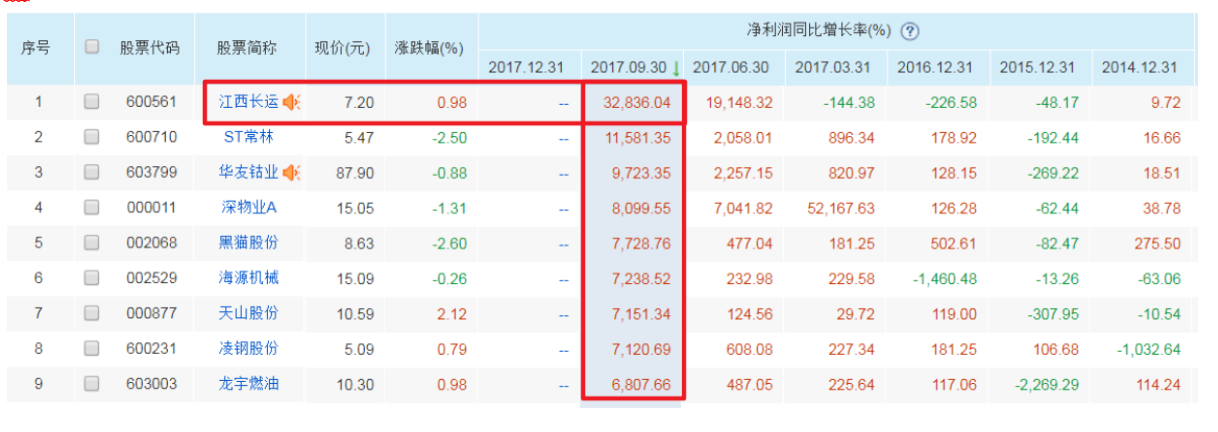以沪深300成分股的pe值为原始数据，向大家阐述MAD、 3σ、百分位法。
import numpy as np
import pandas as pd
import math
from statsmodels import regression
import statsmodels.api as sm
import matplotlib.pyplot as plt

date='20180125'
stock=get_index_stocks('000300.SH',date)
q = query(
valuation.symbol,
valuation.pe_ttm,
valuation.current_market_cap
).filter(valuation.symbol.in_(stock))
print(type(q))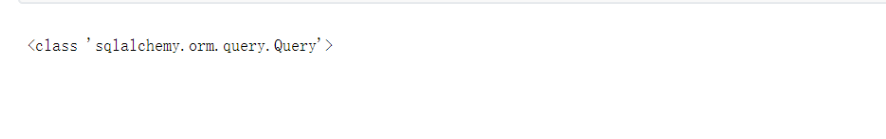data = get_fundamentals(q,date=date)
print(type(data))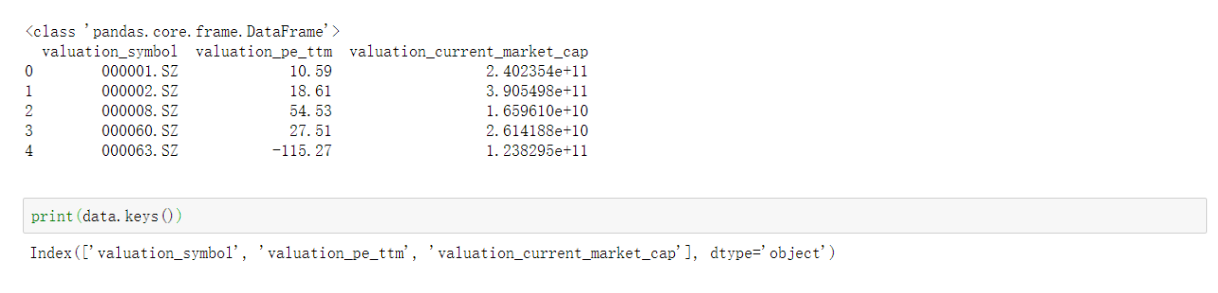# 修改索引名字
data.columns = [['symbol','pe_ratio','market_cap']]
print(data.keys())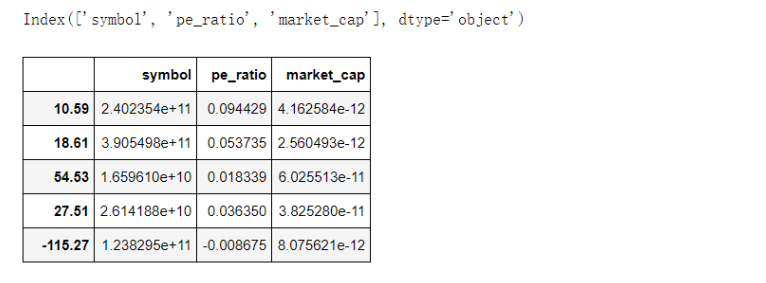data = data.set_index(data.symbol.values)
print(data.keys())
del data['symbol']
data['1/PE'] = 1/data['pe_ratio']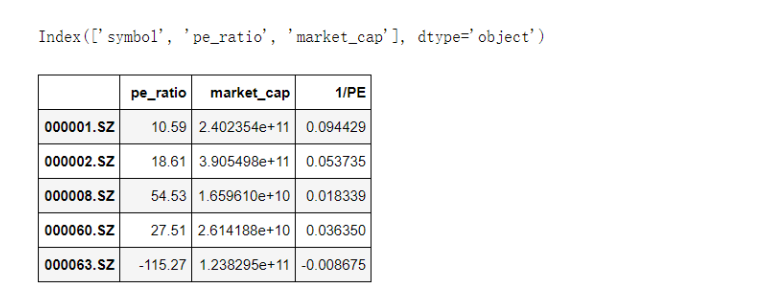将1/PE的数据分布，运用绘图函数展示出来：

1/PE就市盈率的倒数，即每股收益相对你付出每股价格的投资回报率。20倍PE则说明如果不增长的前提下，每股收益相对于你付出的价格的回报率是5%，20年回本。所以，1/PE是从投资回报率以及投资回收年限的角度来分析。一般来说，如果单纯的每年5%的回报率，大家是不满意的，更多人还是因为公司会不断发展，利润会不断增加，那么回报率也会增加，投资回收年限减少。

fig = plt.figure(figsize = (20, 8))
ax = data['1/PE'].plot.kde(label = 'Original_PE')
ax.legend()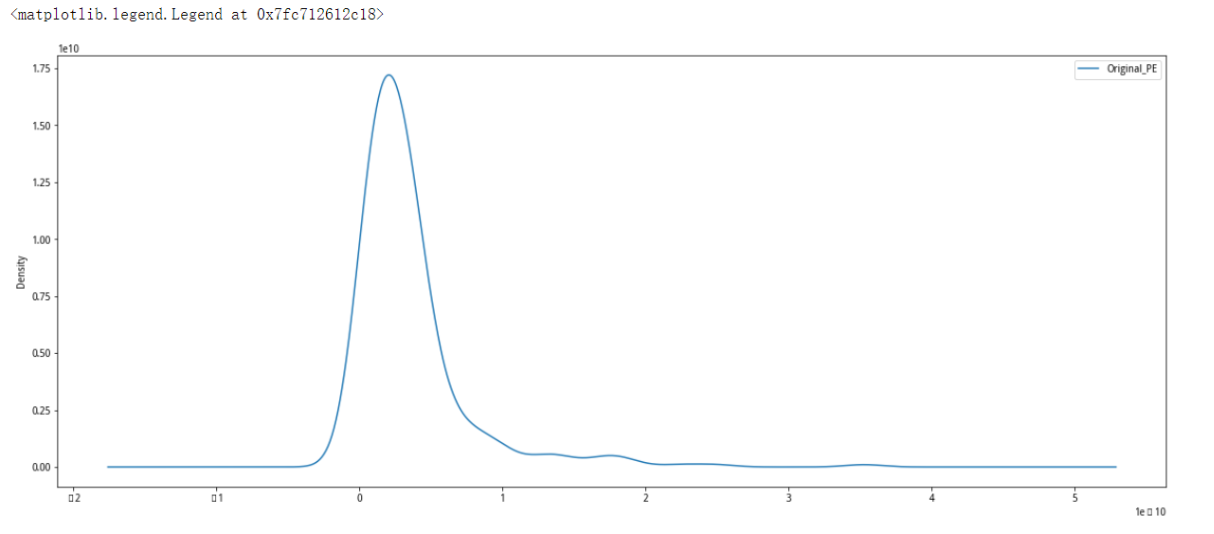第一步，找出所有因子的中位数 Xmedian 　　　第二步：得到每个因子与中位数的绝对偏差值 Xi−Xmedian 　　　第三步：得到绝对偏差值的中位数 MAD 　　　第四步：确定参数 n，从而确定合理的范围为 [Xmedian−nMAD,Xmedian+nMAD]，并针对超出合理范围的因子值做如下的调整：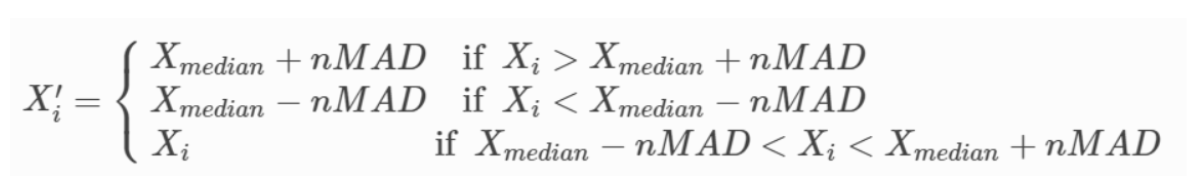def filter_extreme_MAD(series,n): #MAD:中位数去极值
median = series.quantile(0.5)
new_median = ((series - median).abs()).quantile(0.50)
max_range = median + n*new_median
min_range = median - n*new_median
return np.clip(series,min_range,max_range)

fig = plt.figure(figsize = (20, 8))
ax = data['1/PE'].plot.kde(label = 'Original_PE')
ax.legend()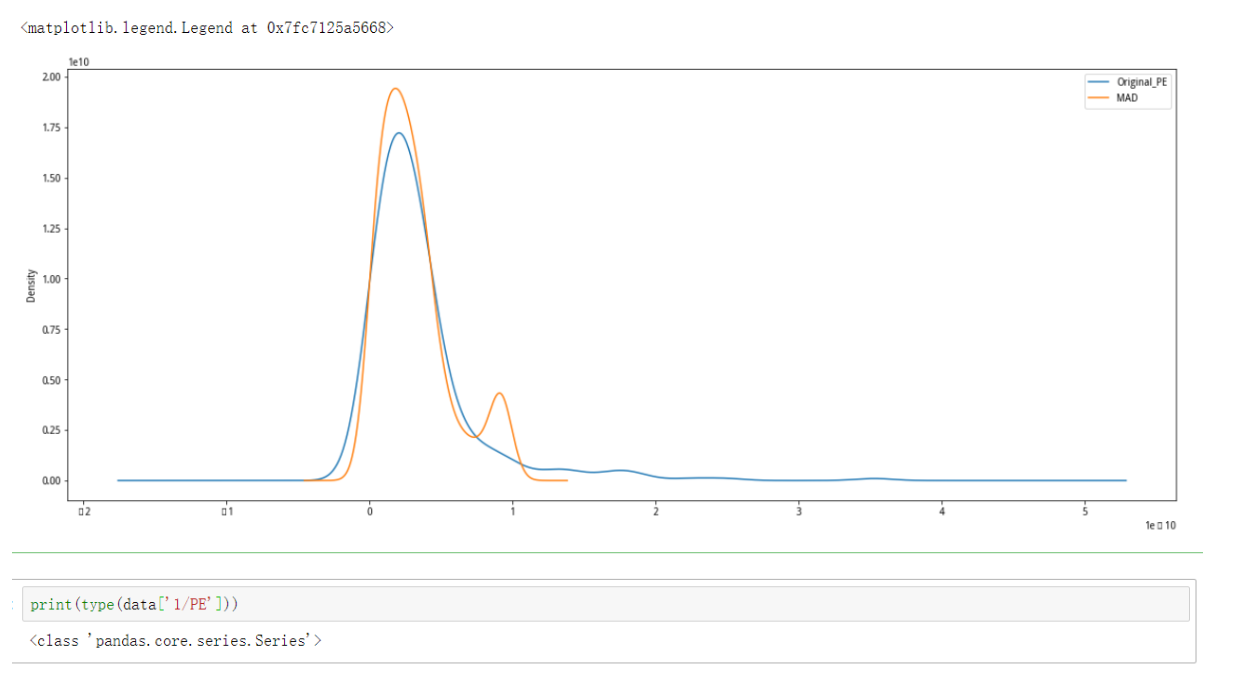1.1.2 3σ法
3σ法又称为标准差法。标准差本身可以体现因子的离散程度，是基于因子的平均值 Xmean而定的。在离群值处理过程中，可通过用 Xmean±nσ来衡量因子与平均值的距离。
标准差法处理的逻辑与MAD法类似: 　　　第一步：计算出因子的平均值与标准差 　　　第二步：确认参数 n（这里选定 n = 3） 　　　第三步：确认因子值的合理范围为 [Xmean−nσ,Xmean nσ]，并对因子值作如下的调整：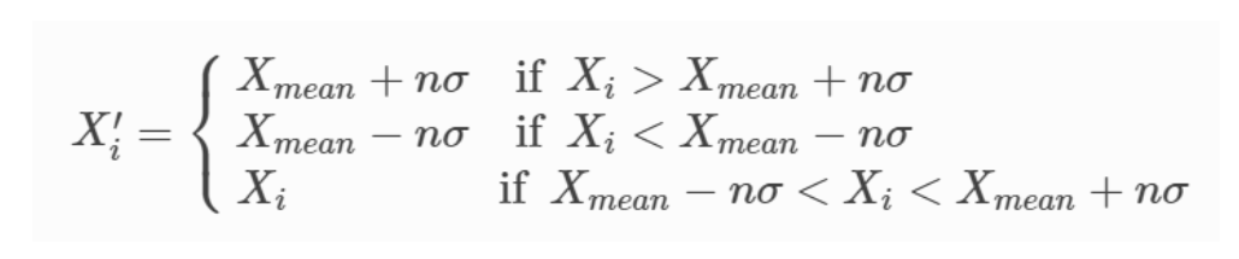3σ代码实现：
def filter_extreme_3sigma(series,n=3): #3 sigma
mean = series.mean()
std = series.std()
max_range = mean + n*std
min_range = mean - n*std
return np.clip(series,min_range,max_range)

对原始数据进行3σ处理后的结果：
fig = plt.figure(figsize = (20, 8))
ax = data['1/PE'].plot.kde(label = 'Original_PE')
ax = filter_extreme_3sigma(data['1/PE']).plot.kde(label = '3sigma')
ax.legend()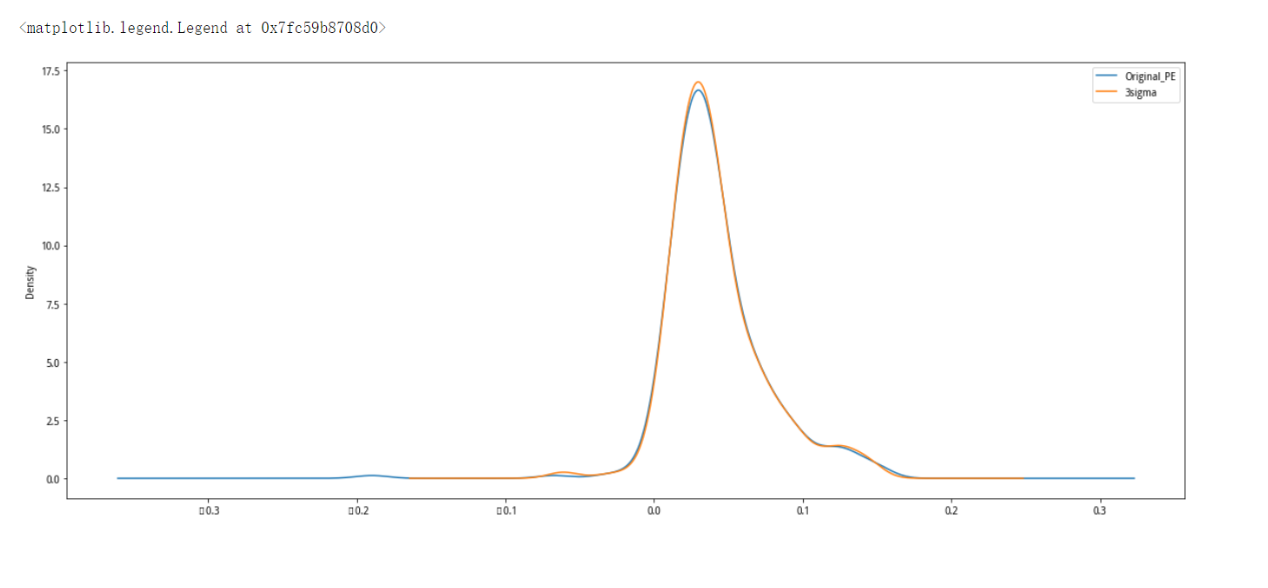1.1.3 百分位法：
百分位法代码实现：
def filter_extreme_percentile(series,min = 0.10,max = 0.90): #百分位法
series = series.sort_values()
q = series.quantile([min,max])
return np.clip(series,q.iloc,q.iloc)

fig = plt.figure(figsize = (20, 8))
ax = data['1/PE'].plot.kde(label = 'Original_PE')
ax = filter_extreme_percentile(data['1/PE']).plot.kde(label = 'Percentile')
ax.legend()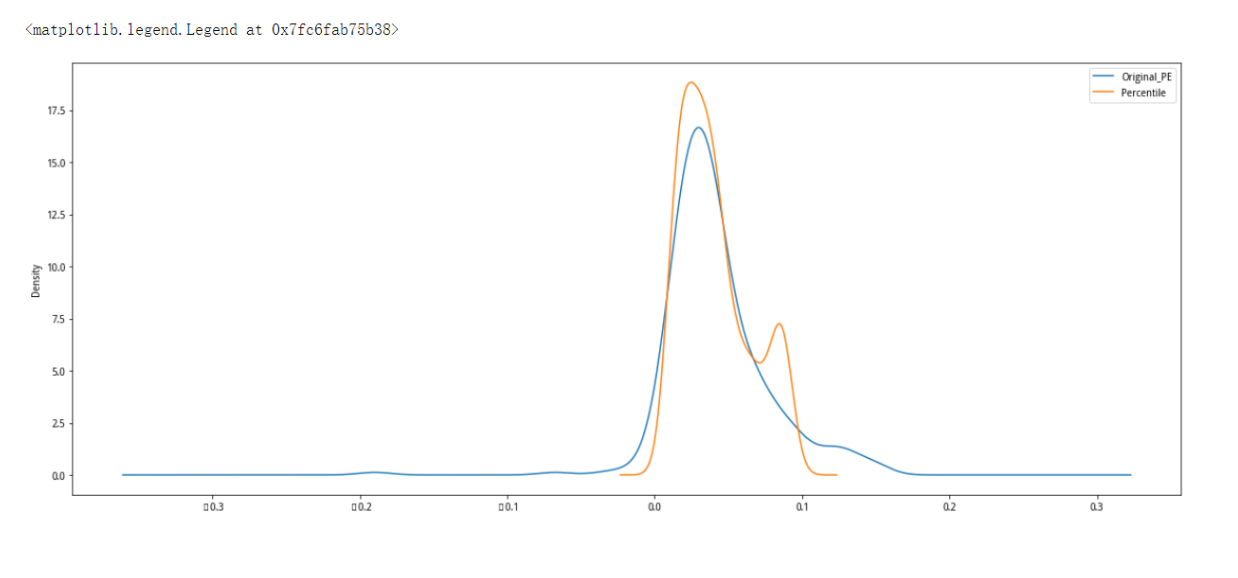1.2 标准化
继续深入的做股票数据分析，假设我们需买入当季净利润同比增长率较高和股息率较高的股票，如果单纯的将两个指标相加，然后取最终值较大的那些股票，那么会面临一个非常严重的问题：两个指标的数值意义不同。上市公司达到股息率10%难于净利润同比增长率10%，一般股息率都小于5%，而净利润增长率则远远超过5%的数值，因此简单的相加，然后选股会淡化股息率指标。
那么这种情况下，数据标准化处理可以解决问题。
标准化在统计学中有一系列含义，一般使用z-score的方法。处理后的数据从有量纲转化为无量纲，使得不同的指标能够进行比较和回归。
z-score的方法介绍
标准化后的数值 = (原始值-单个指标内所有值的均值)/单个指标内所有值的标准差
z-score的方法代码实现：
def standardize_series(series): #原始值法
std = series.std()
mean = series.mean()
return (series-mean)/std

fig = plt.figure(figsize = (20, 8))

new = filter_extreme_3sigma(data['1/PE'])

ax = standardize_series(new).plot.kde(label = 'standard_1')
ax.legend()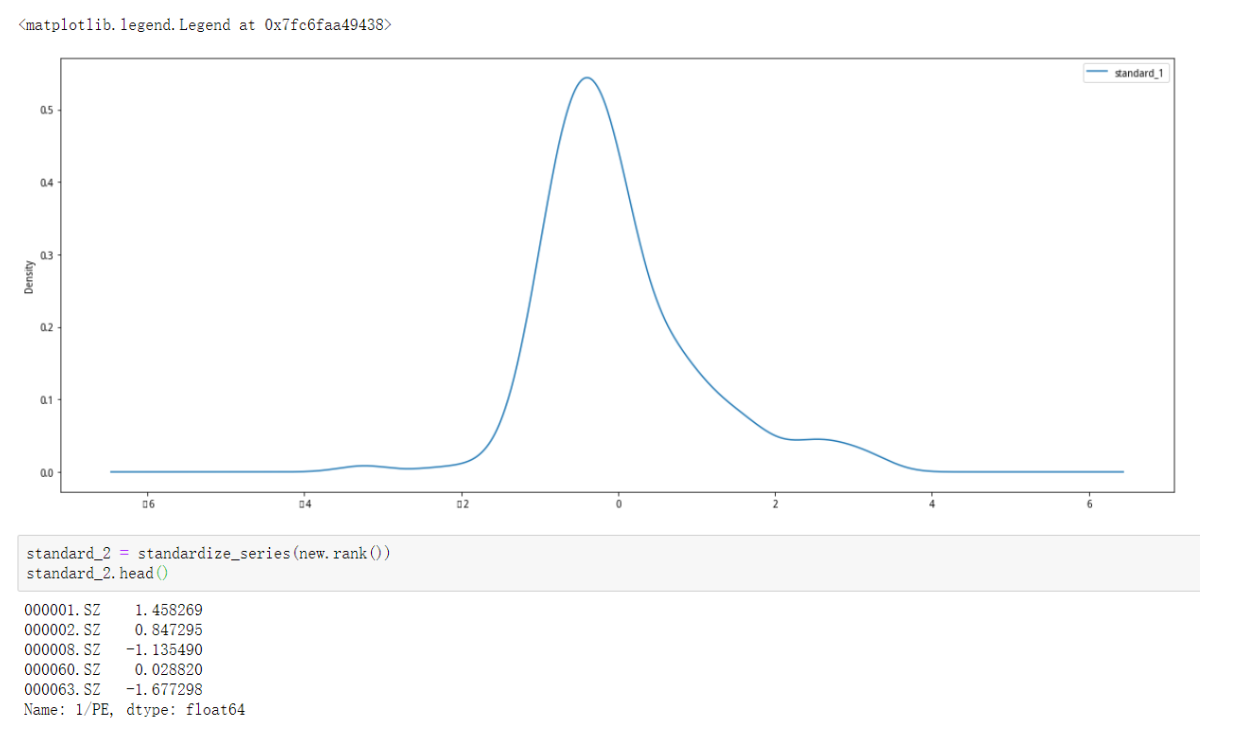展开全文python 数据分析 标准化
• #为什么要做去极值的工作（Why） 在做回归分析的时候，因为过大或过小的数据可能会影响到分析结果，离群值会严重影响因子和收益率之间的相关性估计结果，因此需要对那些离群值进行处理 ## 有哪些去极值的方法...
• 去极值是一个更广泛的概念，极值是异常值的一种，先找出极值（异常值），再去掉极值，算是一个完整的“去极值”过程。 Detect Anomalies 1.Standard Deviation For a data distribution is approximately normal ...Detect MAD 3sigma
• 2. 去极值处理 不是删除，而是拉回正常范围。 2.1 三种方法 分位数去极值 中位数 绝对偏差去极值 正态分布去极值 3.分位数去极值 3.1 中位数 排序后，处于中间位置的那个数。Median 奇数：中间的那个数 偶数：中间...python 量化交易
• 一、去极值 百分位去极值：直接以上下百分位为边界，将边界外数据归为边界上数据，目前行业内一般不使用。 标准化去极值：又称为标准差法。标准差本身可以体现因子的离散程度，是基于因子的平均值 Xmean而定的。在...
• import numpy as np import pandas as pd from pandas import Series def filter_extereme_MAD(series,n): median=series.quantile(0.5) n1_median=((series-median).abs()).quantile(0.50) max_rangef=median+n*...
• #因子去极值及标准化 def normal(a): Func = lambda a:(a-a.mean())/a.std() norm = a.apply(Func) return norm def winsorize(a,limit=3): def func(a): a[a<a.mean()-a.std()*limit] = a.mean() - a.std...
• 导语：一般的数据预处理中常提及到三类处理：去极值、标准化、中性化。我们将向大家讲述这常见的 三种数据处理操作。 一、去极值 在分析上市公司当季净利润同比增长率数据时，我们往往会被其中一些公司的数据干扰...量化投资 python
• 去极值的方法，可以用均值加n倍的方差，来过滤，也可以用中位数加上下范围来过滤。如聚宽就提供了winsorize和winsorize_med等方法。 但我总觉得不合心意，第一，这个过程本来就是需要不断调整参数的，最好能够按照...
• 3 ways to remove outliers from your data https://ocefpaf.github.io/python4oceanographers/blog/2015/03/16/outlier_detection/ ...According to Google Analytics, my post "Dealing ... with spiky datpython threshold
• 异常值是指那些分布明显异于普通分布...正态分布去极值 这种想法的思路来自于正态分布，假设X?N(μ,σ2)，那么： P(|X?μ|&gt;k?σ)=?????0.317,0.046, 0.003,k=1k=2k=3通常把三倍标准差之外的值都视为异常值...python
• 拿到数据后，数据中可能会存在一些超大或极小的值，这些值与其他的值离得较远，显得格格不入，我们称其为离群点，有时也称为异常点。对于这些值，它的存在会导致影响最终的分析结果，带偏...（一）数据去极值 数据去极python 数据分析 tushare
• 指标无量纲化处理时的极值处理法，文件中包含两个.m文件，一个为极大型数据的极值处理法，另一个为极小型数据的极值处理法。使用matlab编程语言实现。
• 主要介绍了python求极值点（波峰波谷）求极值点主要用到了scipy库，本文通过实例代码给大家介绍的非常详细，具有一定的参考借鉴价值,需要的朋友可以参考下...